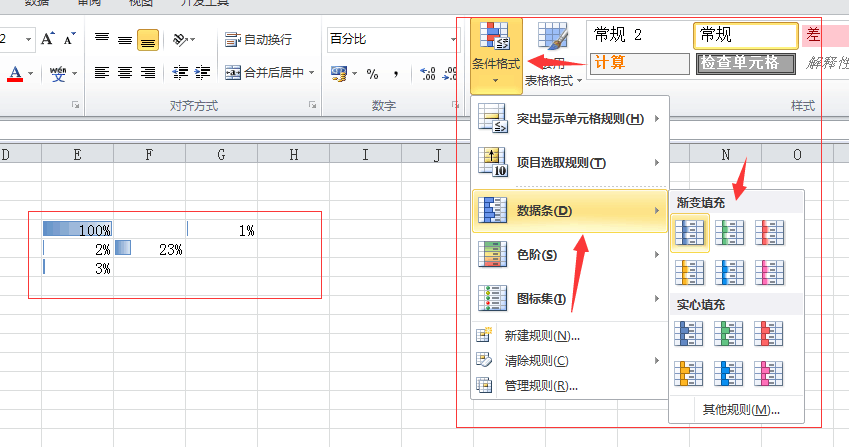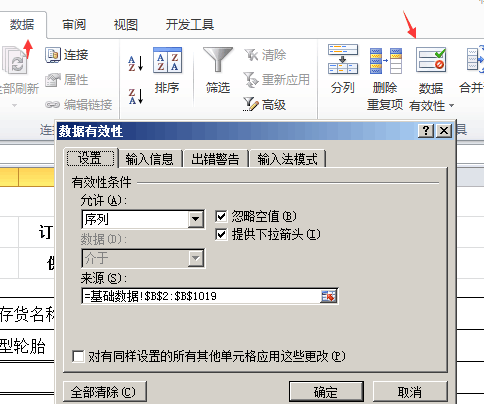# excel使用技巧

=IF(ISNUMBER(FIND(“机械设备”,N2)),”OK”, “Not OK”)01010001 3DA80型轮胎（黑色） 3D40S3.00-10·80 01010002 3DA80型轮胎（红色） 3D40Ss3.00-10·80

=IFERROR(INDEX(基础数据!A:A,MATCH(B6,基础数据!B:B,)),””)

B6 代表你当前单元格的数据

vba代码

Function phonenum(arr As String) As String
num = “3031323334353637383945474849505152535455565758596671727375767778798081828384858687888998”
For i = 1 To Len(arr) – Len(Replace(arr, “,”, “”))
For j = 1 To 100 Step 2
If Mid(Val(arr), 1, 3) = “1” & Mid(num, j, 2) Then
phonenum = phonenum & IIf(phonenum = “”, “”, “,”) & Val(arr)
Exit For
End If
Next
arr = Trim(Mid(arr, InStr(arr, “,”) + 1, 999))
Next
End Function

A1代表需要获取的电话号码单元格

For i = 1 To Len(arr) – Len(Replace(arr, “,”, “”))

num = “30313233343536373839605658”解释

130, 131, 132….

For j循环 后 Step 2

134 135 136 137 138 139 147 150 151 152 157 158 159 172 178 182 183 184 187 188 198

130 131 132 145 155 156 166 171 175 176 185 186

133 149 153 173 177 180 181 189 199

170

Function CellNum\$(str) ‘自定义函数：手机号码提取
Set reg = CreateObject(“vbscript.regexp”)
reg.Global = True
reg.Pattern = “(?:^|\D+)(1(3\d|4|5[0-35-9]|7[0136-8]|8\d)\d{8})(?=(\D+|\$))” ‘精确验证：位数不符、号段不符（按2017年12月手机号段）
Set mas = reg.Execute(str)
For j = 1 To mas.Count
CellNum = CellNum & “;” & mas(j – 1).submatches(0)
Next
CellNum = Mid(CellNum, 2)
End Function